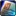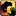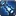Attack speed is a secondary attribute that describes the delay between auto-attacks, measured in seconds. It is the reciprocal of the actual rate of attack; a higher attack speed means that you will attack less frequently, not more frequently. Essentially, it can be considered the "cooldown" between auto-attacks by the given weapon. It is a function of weapon speed and haste.

Attack speed can be found in two places on your character sheet - under Melee and Ranged. Melee attack speed is determined by melee haste, and modifies the speed of your melee auto-attacks, while Ranged attack speed is determined by ranged haste, and affects the speed of your ranged auto-attacks. The same rules and calculations apply to each.

## Attack speed calculation

`Attack speed = base weapon speed / ((haste percentage / 100) + 1)`

Like your attack speed, your melee and ranged haste can be found on your character sheet in the appropriate section. This percentage reflects a combination of all current haste effects applicable to your attacks; don't forget to apply melee haste for melee attack speed calculations, and ranged haste for ranged attack speed.

When calculating your own haste effects, you can either calculate the total haste percentage first, or perform the calculation separately for each haste source. Haste sources stack multiplicatively, except for haste rating, which is combined into a single percentage before being multiplied with the others.

### Example

Melee weapon speed = `1.8`
Slice and Dice = `40%`
Blade Flurry = `20%`
JuJu Flurry = `3%`
`Attack_speed = 1.8 / ( (40 / 100) + 1 ) = 1.2857`
`Attack_speed = 1.2857 / ( (20 / 100) + 1 ) = 1.0714`
`Attack_speed = 1.0714 / ( (3 / 100) + 1 ) = 1.0402`

Alternatively, multiply the haste sources at the start:

`1.4 * 1.2 * 1.03 = 1.7304 = 73.04% `
`1.8 / 1.7304 = 1.0402`

So with all 3 speed buffs going the melee attack speed is now 1.0402

`1.8 / 1.0402 = 1.73 = 73% increased attack speed`

Or, in attacks per minute:

`57.6812 / 33.3417 = 1.73 = 73% more attacks per minute`

Note: Blizzard rounds to 4 significant digits.

Basically, all of the calculations are reciprocal operations and are inverted from how you would expect to see them.

Blizzard applies attack speed buffs multiplicatively; in this example the buffs provide 73% increase, because 40% increase times 20% increase times 3% increase is 1.4 * 1.2 * 1.03 = 1.7304.

If you flip the numerator and denominator, you get your number of attacks per second, meaning that a percentage increase in attack speed has the final result of increasing your damage per second by the same percentage, along with an added bonus of increasing the number of ability procs (from weapons ([Landslide]) or skills and talents ([Go for the Throat],[Might of the Frozen Wastes]).

A quick way to determine resultant attack speed, without applying the formula for each increase, is the following:

[current attack speed] / (increase1 * increase2 * increase3...etc)

Example:

1.8 / (1.40 * 1.20 * 1.03) = 1.0402

## Attack speed and DPS

It must be remembered that attack speed's significance is inverse to the usual meaning of 'speed' - in this case, higher speed means attacking more slowly/less frequently, while lower speed means attacking more quickly/more frequently. Attack speed describes the length of time between a player's auto-attacks. Effects that "slow" opponents' attacks actually increase their attack speed.

`DPS = weapon damage / attack speed`

### Example

Weapon damage = 7600
Attack speed = 3.43
`DPS = 7600 / 3.43 = 2216`

While each attack may deal 7600 damage, because an auto-attack will only occur once every 3.43 seconds, the weapon's DPS is 2216.